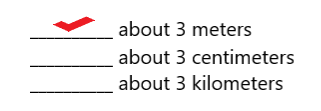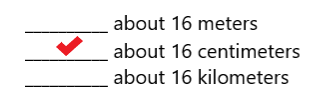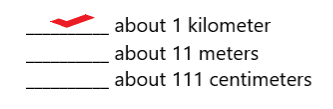# McGraw Hill Math Grade 4 Chapter 10 Lesson 1 Answer Key Metric Units of Length

Practice the questions of McGraw Hill Math Grade 4 Answer Key PDF Chapter 10 Lesson 1 Metric Units of Length to secure good marks & knowledge in the exams.

## McGraw-Hill Math Grade 4 Answer Key Chapter 10 Lesson 1 Metric Units of Length

Solve

Place a check mark next to the best answer.

Question 1.
About how long is a bee?Explanation:
The bee is about 1 centimeter long.

Question 2.
About how long is a car?Explanation:
The car is about 3 meters long.

Question 3.
About how long is a new pencil?Explanation:
The new pencil is about 16 centimeters long.

Question 4.
About how long is a city block?Explanation:
The city block is about 1 kilometer long.

Multiply to find each missing number.

Question 5.
30 km =____________ m
30 km = 30000 m

Explanation:
1 km = 1000 m
30 km = 30 x 1000 m
= 30000 m
So, 30 km = 30000 m.

Question 6.
5 m = ____________ cm
5 m = 500 cm

Explanation:
1 m = 100 cm
5 m = 5 x 100 cm
= 500 cm
So, 5 m = 500 cm.

Question 7.
8 km = ___________ m